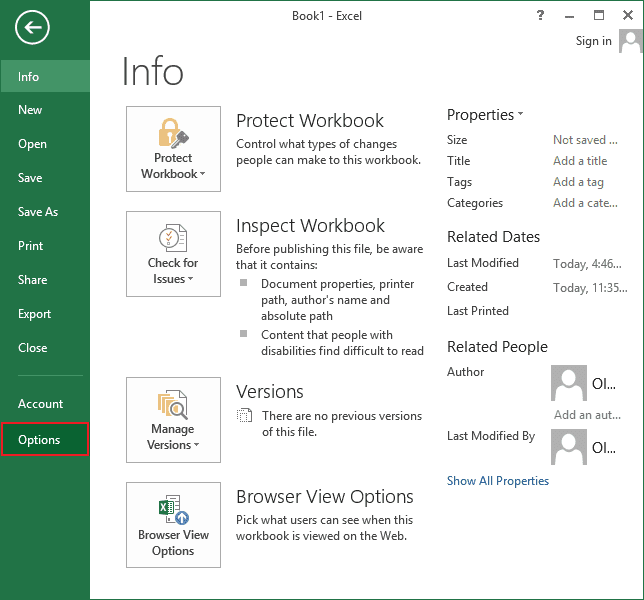# Recalculating the worksheet

Excel
If you are working with huge workbooks that contain a very large number of formulas, functions, and data, you can turn off the automatic recalculation.

Turning off the automatic calculation feature also means that you must remember to manually recalculate the values in the worksheet before you print. To change the recalculation setting, take the following steps:

1.   On the Home, click Excel Options:2.   In the Formulas category, under Calculation options, select one of the following Workbook Calculation options:• Automatic - This is the default setting. It recalculates the entire workbook each time you edit or enter a formula.
• Automatic except for data tables - This automatically recalculates everything except formulas in a data table (data tables are used to provide a range of data for one formula and function and are used for an advanced Excel feature called "What If Analysis").
• Manual - This option tells Excel to recalculate only when you say so. To recalculate manually, press the F9 key. When this option is selected, you can turn the Recalculate workbook before saving option off or on.

3.   Click OK.